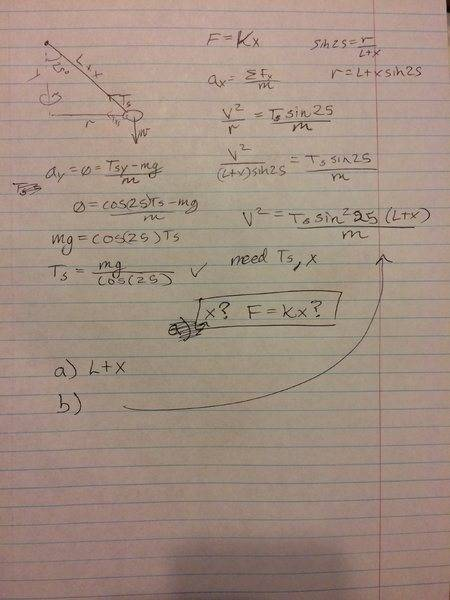# Need help with spring problem

• Ruskointhehizzy
In summary, the ball moves at .73m/s when it is attached to the spring and the spring has a spring constant of 35.0 N/m.f

## Homework Statement

A ball of mass 0.250 kg is attached to a spring with spring constant 35.0 N/m and relaxed length 0.200 m. The other end of the spring is attached to a vertical pole. The ball is set into circular motion in the horizontal plane with the spring making an angle 25degrees with the pole.
a) find the length of the spring during this motion.
b) find the velocity of the ball during this motion.

## The Attempt at a Solution

I have set it up to find v, but am stuck on how to find x. Thank you very much.#### Attachments

•ephphatha16
##T_s = kx## should be the missing equation. Simply the equation of the spring extension.

Some comments what you calculate where would make it easier to follow.

•ephphatha16 and Ruskointhehizzy
##T_s = kx## should be the missing equation. Simply the equation of the spring extension.

Some comments what you calculate where would make it easier to follow.
Okay I thought so but wasn't sure. I will be sure to include comments from here on forth. Thank you very much! I love this site you guys are the best

•mfb
I’d just like to point out, “L+x” is not a final answer to part a). The ‘extension’ x is a variable introduced by you, not given in the problem.

If you use T_s = kx in your vertical equation (on the left) then you can express x in terms of known variables, and then you could calculate the length explicitly.

•ephphatha16
I’d just like to point out, “L+x” is not a final answer to part a). The ‘extension’ x is a variable introduced by you, not given in the problem. .
I set Tx to kx and found x using known terms and it gave me .077 which is correct.
using the tension I found and the new length I was able to calculate the velocity at .73m/s which is also correct. Thanks for pointing that out.

thanks for the help everyone! I really enjoyed this problem.